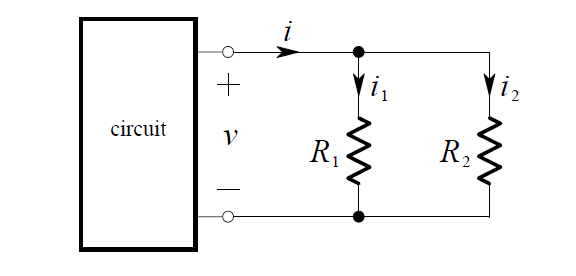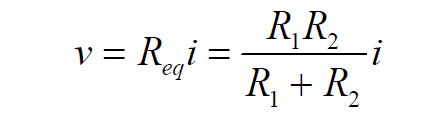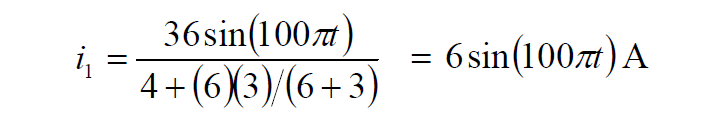# Current Divider Rule

It can be quite useful to determine how a current entering two parallel resistors “divides” between them.

Consider the circuit shown below:We replace the parallel connection of R1   and R2  by its equivalent resistance.

Thus, Ohm’s Law gives:By application of Ohm’s Law again, the current in R1  is i1  = v R1 and thus:Similarly, the current in R2 is :These equations describe how the current is divided between the resistors. Because of this, a pair of resistors in parallel is often called a current divider. Note that a larger amount of current will exist in the smaller resistor – thus current tends to take the path of least resistance!

Example:

We want to find the current i in the circuit below:The total current delivered by the source is:Therefore the desired current is: